# Spiking Neural Systems

##### Daniel Müller-Komorowska

This is an introduction to spiking neural systems with Julia's DifferentialEquations package. We will cover three different models: leaky integrate-and-fire, Izhikevich, and Hodgkin-Huxley. Finally we will also learn about two mechanisms that simulate synaptic inputs like real neurons receive them. The alpha synapse and the Tsodyks-Markram synapse. Let's get started with the leaky integrate-and-fire (LIF) model.

## The Leaky Integrate-and-Fire Model

The LIF model is an extension of the integrate-and-fire (IF) model. While the IF model simply integrates input until it fires, the LIF model integrates input but also decays towards an equilibrium potential. This means that inputs that arrive in quick succession have a much higher chance to make the cell spike as opposed to inputs that are further apart in time. The LIF is a more realistic neuron model than the IF, because it is known from real neurons that the timing of inputs is extremely relevant for their spiking.

The LIF model has five parameters, gL, EL, C, Vth, I and we define it in the lif(u, p, t) function.

using DifferentialEquations
using Plots
gr()

function lif(u,p,t);
gL, EL, C, Vth, I = p
(-gL*(u-EL)+I)/C
end

lif (generic function with 1 method)


Our system is described by one differential equation: (-gL*(u-EL)+I)/C, where u is the voltage, I is the input, gL is the leak conductance, EL is the equilibrium potential of the leak conductance and C is the membrane capacitance. Generally, any change of the voltage is slowed down (filtered) by the membrane capacitance. That's why we divide the whole equation by C. Without any external input, the voltage always converges towards EL. If u is larger than EL, u decreases until it is at EL. If u is smaller than EL, u increases until it is at EL. The only other thing that can change the voltage is the external input I.

Our lif function requires a certain parameter structure because it will need to be compatible with the DifferentialEquations interface. The input signature is lif(u, p, t) where u is the voltage, p is the collection of the parameters that describe the equation and t is time. You might wonder why time does not show up in our equation, although we need to calculate the change in voltage with respect to time. The ODE solver will take care of time for us. One of the advantages of the ODE solver as opposed to calculating the change of u in a for loop is that many ODE solver algorithms can dynamically adjust the time step in a way that is efficient and accurate.

One crucial thing is still missing however. This is supposed to be a model of neural spiking, right? So we need a mechanism that recognizes the spike and hyperpolarizes u in response. For this purpose we will use callbacks. They can make discontinuous changes to the model when certain conditions are met.

function thr(u,t,integrator)
integrator.u > integrator.p
end

function reset!(integrator)
integrator.u = integrator.p
end

threshold = DiscreteCallback(thr,reset!)
current_step= PresetTimeCallback([2,15],integrator -> integrator.p += 210.0)
cb = CallbackSet(current_step,threshold)

DiffEqBase.CallbackSet{Tuple{},Tuple{DiffEqBase.DiscreteCallback{DiffEqCall
backs.var"#61#64"{Array{Int64,1}},DiffEqCallbacks.var"#62#65"{Main.##WeaveS
andBox#253.var"#1#2"},DiffEqCallbacks.var"#63#66"{typeof(DiffEqBase.INITIAL
IZE_DEFAULT),Bool,Array{Int64,1},Main.##WeaveSandBox#253.var"#1#2"}},DiffEq
Base.DiscreteCallback{typeof(Main.##WeaveSandBox#253.thr),typeof(Main.##Wea
veSandBox#253.reset!),typeof(DiffEqBase.INITIALIZE_DEFAULT)}}}((), (DiffEqB
ase.DiscreteCallback{DiffEqCallbacks.var"#61#64"{Array{Int64,1}},DiffEqCall
backs.var"#62#65"{Main.##WeaveSandBox#253.var"#1#2"},DiffEqCallbacks.var"#6
3#66"{typeof(DiffEqBase.INITIALIZE_DEFAULT),Bool,Array{Int64,1},Main.##Weav
eSandBox#253.var"#1#2"}}(DiffEqCallbacks.var"#61#64"{Array{Int64,1}}([2, 15
]), DiffEqCallbacks.var"#62#65"{Main.##WeaveSandBox#253.var"#1#2"}(Main.##W
eaveSandBox#253.var"#1#2"()), DiffEqCallbacks.var"#63#66"{typeof(DiffEqBase
.INITIALIZE_DEFAULT),Bool,Array{Int64,1},Main.##WeaveSandBox#253.var"#1#2"}
(DiffEqBase.INITIALIZE_DEFAULT, true, [2, 15], Main.##WeaveSandBox#253.var"
#1#2"()), Bool[1, 1]), DiffEqBase.DiscreteCallback{typeof(Main.##WeaveSandB
ox#253.thr),typeof(Main.##WeaveSandBox#253.reset!),typeof(DiffEqBase.INITIA
LIZE_DEFAULT)}(Main.##WeaveSandBox#253.thr, Main.##WeaveSandBox#253.reset!,
DiffEqBase.INITIALIZE_DEFAULT, Bool[1, 1])))


Our condition is thr(u,t,integrator) and the condition kicks in when integrator.u > integrator.p where p is our threshold parameter Vth. Our effect of the condition is reset!(integrator). It sets u back to the equilibrium potential p. We then wrap both the condition and the effect into a DiscreteCallback called threshold. There is one more callback called PresetTimeCallback that is particularly useful. This one increases the input p at t=2 and t=15 by 210.0. Both callbacks are then combined into a CallbackSet. We are almost done to simulate our system we just need to put numbers on our initial voltage and parameters.

u0 = -75
tspan = (0.0, 40.0)
# p = (gL, EL, C, Vth, I)
p = [10.0, -75.0, 5.0, -55.0, 0]

prob = ODEProblem(lif, u0, tspan, p, callback=cb)

ODEProblem with uType Int64 and tType Float64. In-place: false
timespan: (0.0, 40.0)
u0: -75


Our initial voltage is u0 = - 75, which will be the same as our equilibrium potential, so we start at a stable point. Then we define the timespan we want to simulate. The time scale of the LIF as it is defined conforms roughly to milliseconds. Then we define our parameters as p = [10.0, -75.0, 5.0, -55.0, 0]. Remember that gL, EL, C, Vth, I = p. Finally we wrap everything into a call to ODEProblem. Can't forget the CallbackSet. With that our model is defined. Now we just need to solve it with a quick call to solve.

sol = solve(prob)

retcode: Success
Interpolation: automatic order switching interpolation
t: 153-element Array{Float64,1}:
0.0
9.999999999999999e-5
0.0010999999999999998
0.011099999999999997
0.11109999999999996
1.1110999999999995
2.0
2.0
2.6300346673750097
2.9226049547524595
⋮
38.34157935968204
38.78215179003683
38.78215179003683
39.222724173706894
39.222724173706894
39.6632965982261
39.6632965982261
40.0
40.0
u: 153-element Array{Float64,1}:
-75.0
-75.0
-75.0
-75.0
-75.0
-75.0
-75.0
-75.0
-59.978080111690375
-57.32999167299642
⋮
-75.0
-50.40489310815222
-75.0
-50.404894730067554
-75.0
-50.404893310891545
-75.0
-54.419318668318546
-75.0


First of all the solve output tells us if solving the system generally worked. In this case we know it worked because the return code (retcode) says Success. Then we get the numbers for the timestep and the solution to u. The raw numbers are not super interesting to let's plot our solution.

plot(sol)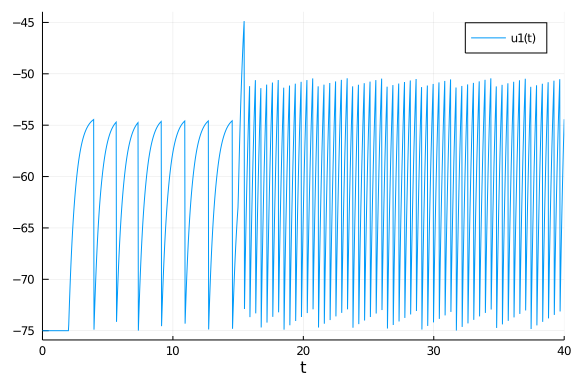We see that the model is resting at -75 while there is no input. At t=2 the input increases by 210 and the model starts to spike. Spiking does not start immediately because the input first has to charge the membrane capacitance. Notice how once spiking starts it very quickly becomes extremely regular. Increasing the input again at t=15 increases firing as we would expect but it is still extremely regular. This is one of the features of the LIF. The firing frequency is regular for constant input and a linear function of the input strength. There are ways to make LIF models less regular. For example we could use certain noise types at the input. We could also simulate a large number of LIF models and connect them synaptically. Instead of going into those topics, we will move on to the Izhikevich model, which is known for its ability to generate a large variety of spiking dynamics during constant inputs.

## The Izhikevich Model

The Izhikevich model is a two-dimensional model of neuronal spiking. It was derived from a bifurcation analysis of a cortical neuron. Because it is two-dimensional it can generate much more complex spike dynamics than the LIF model. The kind of dynamics depend on the four parameters and the input a, b, c, d, I = p. All the concepts are the same as above, expect for some minor changes to our function definitions to accomodate for the second dimension.

#Izhikevichch Model
using DifferentialEquations
using Plots

function izh!(du,u,p,t);
a, b, c, d, I = p

du = 0.04*u^2+5*u+140-u+I
du = a*(b*u-u)
end

izh! (generic function with 1 method)


This is our Izhikevich model. There are two important changes here. First of all, note the additional input parameter du. This is a sequence of differences. du corresponds to the voltage (the first dimension of the system) and du corresponds to the second dimension. This second dimension is called u in the original Izhikevich work amnd it makes the notation a little annoying. In this tutorial I will generally stick to Julia and DifferentialEquations conventions as opposed to conventions of the specific models and du is commonly used. We will never define du ourselves outside of the function but the ODE solver will use it internally. The other change here is the ! after our function name. This signifies that du will be preallocated before integration and then updated in-place, which saves a lot of allocation time. Now we just need our callbacks to take care of spikes and increase the input.

function thr(u,t,integrator)
integrator.u >= 30
end

function reset!(integrator)
integrator.u = integrator.p
integrator.u += integrator.p
end

threshold = DiscreteCallback(thr,reset!)
current_step= PresetTimeCallback(50,integrator -> integrator.p += 10)
cb = CallbackSet(current_step,threshold)

DiffEqBase.CallbackSet{Tuple{},Tuple{DiffEqBase.DiscreteCallback{DiffEqCall
backs.var"#61#64"{Int64},DiffEqCallbacks.var"#62#65"{Main.##WeaveSandBox#25
3.var"#3#4"},DiffEqCallbacks.var"#63#66"{typeof(DiffEqBase.INITIALIZE_DEFAU
LT),Bool,Int64,Main.##WeaveSandBox#253.var"#3#4"}},DiffEqBase.DiscreteCallb
ack{typeof(Main.##WeaveSandBox#253.thr),typeof(Main.##WeaveSandBox#253.rese
t!),typeof(DiffEqBase.INITIALIZE_DEFAULT)}}}((), (DiffEqBase.DiscreteCallba
ck{DiffEqCallbacks.var"#61#64"{Int64},DiffEqCallbacks.var"#62#65"{Main.##We
aveSandBox#253.var"#3#4"},DiffEqCallbacks.var"#63#66"{typeof(DiffEqBase.INI
TIALIZE_DEFAULT),Bool,Int64,Main.##WeaveSandBox#253.var"#3#4"}}(DiffEqCallb
acks.var"#61#64"{Int64}(50), DiffEqCallbacks.var"#62#65"{Main.##WeaveSandBo
x#253.var"#3#4"}(Main.##WeaveSandBox#253.var"#3#4"()), DiffEqCallbacks.var"
#63#66"{typeof(DiffEqBase.INITIALIZE_DEFAULT),Bool,Int64,Main.##WeaveSandBo
x#253.var"#3#4"}(DiffEqBase.INITIALIZE_DEFAULT, true, 50, Main.##WeaveSandB
ox#253.var"#3#4"()), Bool[1, 1]), DiffEqBase.DiscreteCallback{typeof(Main.#
#WeaveSandBox#253.thr),typeof(Main.##WeaveSandBox#253.reset!),typeof(DiffEq
Base.INITIALIZE_DEFAULT)}(Main.##WeaveSandBox#253.thr, Main.##WeaveSandBox#
253.reset!, DiffEqBase.INITIALIZE_DEFAULT, Bool[1, 1])))


One key feature of the Izhikevich model is that each spike increases our second dimension u by a preset amount p. Between spikes u decays to a value that depends on p and p and the equilibrium potential p. Otherwise the code is not too different from the LIF model. We will again need to define our parameters and we are ready to simulate.

p = [0.02, 0.2, -50, 2, 0]
u0 = [-65, p*-65]
tspan = (0.0, 300)

prob = ODEProblem(izh!, u0, tspan, p, callback=cb)

ODEProblem with uType Array{Float64,1} and tType Float64. In-place: true
timespan: (0.0, 300.0)
u0: [-65.0, -13.0]

sol = solve(prob);
plot(sol, vars=1)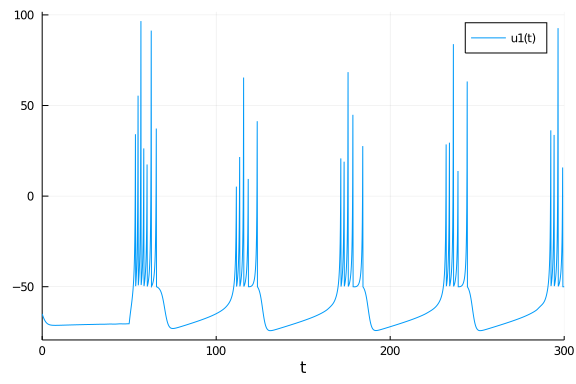This spiking type is called chattering. It fires with intermittent periods of silence. Note that the input starts at t=50 and remain constant for the duration of the simulation. One of mechanisms that sustains this type of firing is the spike induced hyperpolarization coming from our second dimension, so let's look at this variable.

plot(sol, vars=2)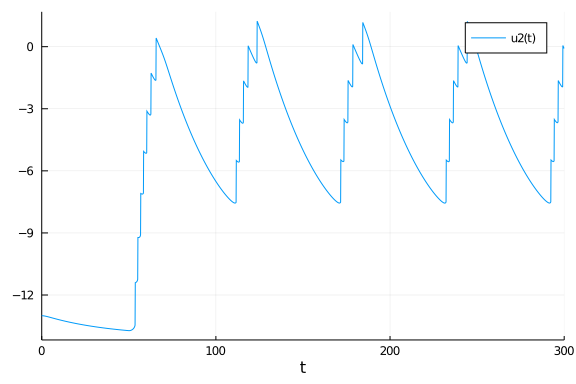Our second dimension u increases with every spike. When it becomes too large, the system cannot generate another spike until u has decayed to a value small enough that spiking can resume. This process repeats. In this model, spiking is no longer regular like it was in the LIF. Here we have two frequencies, the frequency during the spiking state and the frequency between spiking states. The LIF model was dominated by one single frequency that was a function of the input strength. Let's see if we can generate another spiking type by changing the parameters.

p = [0.02, 0.2, -65, 8, 0]
u0 = [-65, p*-65]
tspan = (0.0, 300)

prob = ODEProblem(izh!, u0, tspan, p, callback=cb)
sol = solve(prob);
plot(sol, vars=1)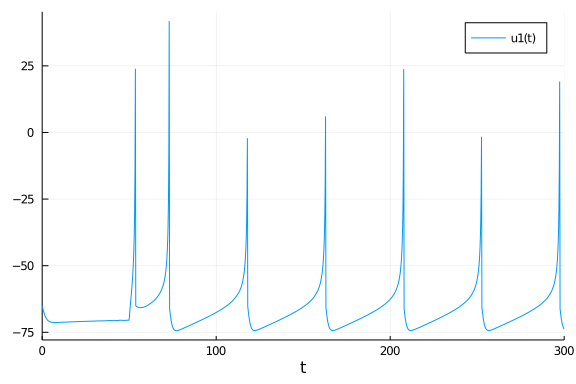This type is called regularly spiking and we created it just by lowering p and increasing p. Note that the type is called regularly spiking but it is not instantaneously regular. The instantenous frequency is higher in the beginning. This is called spike frequency adaptation and is a common property of real neurons. There are many more spike types that can be generated. Check out the original Izhikevich work and create your own favorite neuron!

## Hodgkin-Huxley Model

The Hodgkin-Huxley (HH) model is our first biophysically realistic model. This means that all parameters and mechanisms of the model represent biological mechanisms. Specifically, the HH model simulates the ionic currents that depolarize and hyperpolarize a neuron during an action potential. This makes the HH model four-dimensional. Let's see how it looks.

using DifferentialEquations
using Plots

# Potassium ion-channel rate functions
alpha_n(v) = (0.02 * (v - 25.0)) / (1.0 - exp((-1.0 * (v - 25.0)) / 9.0))
beta_n(v) = (-0.002 * (v - 25.0)) / (1.0 - exp((v - 25.0) / 9.0))

# Sodium ion-channel rate functions
alpha_m(v) = (0.182*(v + 35.0)) / (1.0 - exp((-1.0 * (v + 35.0)) / 9.0))
beta_m(v) = (-0.124 * (v + 35.0)) / (1.0 - exp((v + 35.0) / 9.0))

alpha_h(v) = 0.25 * exp((-1.0 * (v + 90.0)) / 12.0)
beta_h(v) = (0.25 * exp((v + 62.0) / 6.0)) / exp((v + 90.0) / 12.0)

function HH!(du,u,p,t);
gK, gNa, gL, EK, ENa, EL, C, I = p
v, n, m, h = u

du = (-(gK * (n^4.0) * (v - EK)) - (gNa * (m ^ 3.0) * h * (v - ENa)) - (gL * (v - EL)) + I) / C
du = (alpha_n(v) * (1.0 - n)) - (beta_n(v) * n)
du = (alpha_m(v) * (1.0 - m)) - (beta_m(v) * m)
du = (alpha_h(v) * (1.0 - h)) - (beta_h(v) * h)
end

HH! (generic function with 1 method)


We have three different types of ionic conductances. Potassium, sodium and the leak. The potassium and sodium conducance are voltage gated. They increase or decrease depending on the voltage. In ion channel terms, open channels can transition to the closed state and closed channels can transition to the open state. It's probably easiest to start with the potassium current described by gK * (n^4.0) * (EK - v). Here gK is the total possible conductance that we could reach if all potassium channels were open. If all channels were open, n would equal 1 which is usually not the case. The transition from open state to closed state is modeled in alpha_n(v) while the transition from closed to open is in beta_n(v). Because potassium conductance is voltage gated, these transitions depend on v. The numbers in alpha_n; beta_n were calculated by Hodgkin and Huxley based on their extensive experiments on the squid giant axon. They also determined, that n needs to be taken to the power of 4 to correctly model the amount of open channels.

The sodium current is not very different but it has two gating variables, m, h instead of one. The leak conductance gL has no gating variables because it is not voltage gated. Let's move on to the parameters. If you want all the details on the HH model you can find a great description here.

current_step= PresetTimeCallback(100,integrator -> integrator.p += 1)

# n, m & h steady-states
n_inf(v) = alpha_n(v) / (alpha_n(v) + beta_n(v))
m_inf(v) = alpha_m(v) / (alpha_m(v) + beta_m(v))
h_inf(v) = alpha_h(v) / (alpha_h(v) + beta_h(v))

p = [35.0, 40.0, 0.3, -77.0, 55.0, -65.0, 1, 0]
u0 = [-60, n_inf(-60), m_inf(-60), h_inf(-60)]
tspan = (0.0, 1000)

prob = ODEProblem(HH!, u0, tspan, p, callback=current_step)

ODEProblem with uType Array{Float64,1} and tType Float64. In-place: true
timespan: (0.0, 1000.0)
u0: [-60.0, 0.0007906538330645915, 0.08362733690208038, 0.41742979353768533
]


For the HH model we need only one callback. The PresetTimeCallback that starts our input current. We don't need to reset the voltage when it reaches threshold because the HH model has its own repolarization mechanism. That is the potassium current, which activates at large voltages and makes the voltage more negative. The three functions n_inf; m_inf; h_inf help us to find good initial values for the gating variables. Those functions tell us that the steady-state gating values should be for the initial voltage. The parameters were chosen in a way that the properties of the model roughly resemble that of a cortical pyramidal cell instead of the giant axon Hodgkin and Huxley were originally working on.

sol = solve(prob);
plot(sol, vars=1)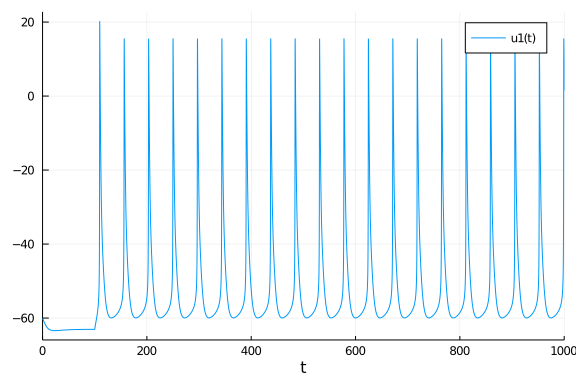That's some good regular voltage spiking. One of the cool things about a biophysically realistic model is that the gating variables tell us something about the mechanisms behind the action potential. You might have seen something like the following plot in a biology textbook.

plot(sol, vars=[2,3,4], tspan=(105.0,130.0))